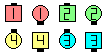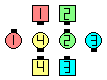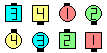Definitions of Square Dance Calls and Concepts

Index -->  Plus  |  A1  |  A2  |  C1  |  C2  |  C3A  |  C3B  |  C4  |  NOL  |
Definitions (Text Only) -->  Plus  |  A1  |  A2  |  C1  |  C2  |  C3A  |  C3B  |  C4  |  NOL  |
 Find call:

anything call \$B\$r:G8e\$N(B Extend \$B\$NA0\$^\$G9T\$C\$F(B, Chain Reaction \$B\$rA4It9T\$\$\$^\$9!%(B

\$BIaDL(B anything call \$B\$O(B Tagging call \$B\$N(B Scoot Back \$B%P%j%(!<%7%g%s\$G\$9!%(B

\$BNc(B,
Flip Reaction: \$BJ?9T\$J(B Wave \$B\$+\$i!%(B Flip Back Centers To A Wave \$B\$r\$7(B, \$BA40w\$G(B Chain Reaction \$B\$r\$7\$^\$9!%(B
Tag Reaction: \$BJ?9T\$J(B Wave \$B\$+\$i!%(B Tag Back Centers To A Wave \$B\$r\$7(B, \$BA40w\$G(B Chain Reaction \$B\$r\$7\$^\$9!%(B
Load The Boat Reaction: From Facing Lines (or R-H Tidal Wave). Load The Boat Centers To A Wave \$B\$r\$7(B, \$BA40w\$G(B Chain Reaction \$B\$r\$7\$^\$9!%(B

\$BTagging CallsScoot Reaction\$B\$NA0(B after Scoot Back Centers To A Wave & \$B30B&\$N?M\$O%+%C%W%k\$K%"%8%c%9%H(B\$B\$N8e(B Chain Reaction\$B\$N8e(B (\$B=*\$o\$j(B)

\$BCm
• Tagging calls \$B\$K\$D\$\$\$F(B, \$B8@\$o\$l\$F\$\$\$J\$\$(B 'Back' (Scoot Back) \$B\$,\$"\$j\$^\$9(B (\$BNc\$(\$P(B, Tag Reaction \$B\$O(B Tag Back Reaction) \$B\$r0UL#\$7\$^\$9!%(B
• \$B30B&\$N?M\$O(B Chain Reaction \$B\$r\$9\$kA0\$K(B, \$BCf\$r8~\$\$\$?%+%C%W%k\$K%"%8%c%9%H\$7\$J\$1\$l\$P\$\$\$1\$^\$;\$s!%(B
• \$B\$3\$N%3!<%k\$G\$O(B, \$B92\$F\$F5^\$KF0\$\$\$F\$O\$\$\$1\$^\$;\$s!%@.8y\$9\$k\$K\$O%A!<%`%o!<%/\$,MW5a\$5\$l\$^\$9!%(BChain Reaction \$B\$r;O\$a\$kA0\$K(B, \$BA40w\$,(B 1/4 tag formation \$B\$r:n\$k\$^\$G(B, \$BBT\$D\$h\$&\$K\$7\$^\$9!%(B
• \$BNc(B:

\$B1~MQ(B: Reaction \$B\$NJQ\$o\$j\$K(B, \$B%3!<%i!<\$O

Chain Reaction [A1,C1] (Lee Kopman 1975): \$BE,@Z\$J0lHL2=\$5\$l\$?(B 1/4 Tag \$B\$+\$i!%(B Very Centers \$B\$H??\$CD>\$0\$K8~\$+\$\$9g\$C\$?30B&\$N?M\$,(B Pass Thru \$B\$r\$9\$k4V\$K(B Center Line \$B\$N(B Ends \$B\$O(B Counter Rotate 1/4 (\$B30B&\$X(B) \$B\$r\$7(B, each side \$B\$N(B Centers \$B\$,(B Hinge, Center 4 \$B\$,(B Star 1/4 \$B\$N4V\$K(B Outsides Trade \$B\$r\$7(B, \$B2q\$C\$??M\$,(B Cast Off 3/4 \$B\$r\$9\$k4V\$KB>\$N30\$O(B move up (Phantom Hourglass Circulate) \$B\$r\$7\$^\$9!%J?9T\$J(B Lines | Waves \$B\$G=*\$o\$j\$^\$9!%(BChoreography for anything ReactionComments? Questions? Suggestions?

https://www.ceder.net/def/anythingreaction.php?language=japan
05-June-2023 05:55:50